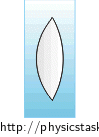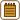## An Air Lens Under WaterA biconvex lens, whose surface is made of very thin glass, is filled with air. Radii of curvature of the lens are $$1 \mathrm{cm}$$ and $$2 \mathrm{cm}$$. We immerse it into water. What is its focal length now? Determine, if it is a converging or a diverging lens.

• #### Notation

 $$r_{1}=1 \mathrm{cm}$$ radius of curvature of the first optical surface $$r_{2}=2 \mathrm{cm}$$ radius of curvature of the second optical surface $$f= ?$$ focal length of the lens
• #### Hint 1

How would you write down that the radii of curvature affect the focal length of the lens? Assume that it is a thin lens.

• #### Hint 2

Express the focal length from the lensmaker’s equation and calculate it.

• #### Hint 3

We have computed the focal length, and now we have to determine whether it is a converging or a diverging lens. According to the look it seems to be a converging lens. Is it really so?

• #### Overall Solution

As we know from the lensmaker’s equation, the radii of curvature affect the focal length of the lens.

We write it for a thin lens: $\frac{1}{f}=\left(\frac{n_{l}}{n_{e}}−1\right)\left(\frac{1}{r_{1}}+\frac{1}{r_{2}}\right),$ where $$n_{l}$$ is the refractive index of the lens and $$n_{e}$$ is the refractive index of the environment.

We express the focal length from the lensmaker’s equation: $f=\frac{1}{\left(\frac{n_{l}}{n_{e}}−1\right)\left(\frac{1}{r_{1}}+\frac{1}{r_{2}}\right)}.$ We adjust the fraction: $f=\frac{1}{\left(\frac{n_{l}−n_{e}}{n_{e}}\right)\left(\frac{r_{2}+r_{1}}{r_{1}r_{2}}\right)},$ $f=\frac{n_{e}r_{1}r_{2}} {\left(n_{l}−n_{e}\right)\left(r_{1}+r_{2}\right)}.$

Numerical solution

We know from the task assignment:

$$r_{1}=1 \mathrm{cm}$$

$$r_{2}=2 \mathrm{cm}$$

$$f= ?$$

We look up the value of the refractive index of air (the lens): $$n_{l}=1{.}00$$ and the refractive index of water (the environment): $$n_{e}=1{.}33$$

We substitute for the values:

$$f=\frac{n_{e}r_{1}r_{2}} {\left(n_{l}−n_{e}\right)\left(r_{1}+r_{2}\right)}= \frac{1{.}33{\cdot} 1\cdot 2} {\left(1{.}00−1{.}33\right)\left(1+2\right)} \mathrm{cm}= \frac{2{.}66} {\left(−0{.}33\right)\cdot 3} \mathrm{cm} \dot=−2{.}7 \mathrm{cm} .$$

The focal length is negative. The sign convention mentioned in Imaging a Bat by a Spherical Mirror and a Converging Lens, Radius of curvature and focal length tells us that if the focal length is negative, then it is a diverging lens. It means that our lens behaves like a diverging lens.

It is so because of the fact that the refractive index of the given biconvex lens is less than the refractive index of the environment. If the refractive index of the lens was greater than the refractive index of the environment, then it would behave like a converging lens.

It is a diverging lens with the focal length of approximately $$2{.}7 \mathrm{cm}$$.